## Saturday, 9 August 2014

### CHAPTER 3 - Worked Out Examples

 Example: 3
 How many lines can we draw that are equally inclined to each of the three coordinate axis?
 Solution: 3
Intuitively, we can expect the answer to be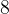$8$, one for each of the$8$ octants. Lets try to derive this answer rigorously.
Assume the direction cosines of the lines to be$l$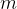$m$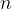$n$. Thus,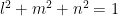${l^2} + {m^2} + {n^2} = 1$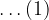$\ldots(1)$
But since the lines are equally inclined to the three axes, we have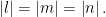$\left| l \right| = \left| m \right| = \left| n \right|.$ This gives using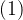$(1)$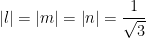$\left| l \right| = \left| m \right| = \left| n \right| = \dfrac{1}{{\sqrt 3 }}$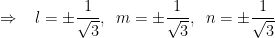$\Rightarrow \,\,\,\,\, l = \pm \dfrac{1}{{\sqrt 3 }},\,\,\,m = \pm \dfrac{1}{{\sqrt 3 }},\,\,\,n = \pm \dfrac{1}{{\sqrt 3 }}$
It is obvious that$8$ combinations of$l$$m$$n$ are possible. Hence,$8$ lines can be drawn which are equally inclined to the axes.
 Example: 4
 Find the direction cosines of the line segment joining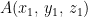$A({x_1},\,{y_1},\,{z_1})$ and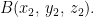$B({x_2},\,{y_2},\,{z_2}).$
 Solution: 4
Refer to Fig –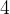$4$. Note that the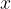$x$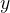$y$ and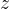$z$ -components of the segment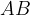$AB$are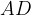$AD$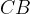$CB$ and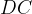$DC$ respectively. If the direction cosines of$AB$ are$l$$m$$n$and the length of$AB$ is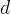$d$, we have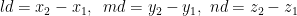$ld = {x_2} - {x_1},\,\,\,md = {y_2} - {y_1},\,\,nd = {z_2} - {z_1}$
Thus, the direction cosines of$AB$ are given by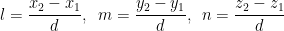${l = \dfrac{{{x_2} - {x_1}}}{d},\,\,\,m = \dfrac{{{y_2} - {y_1}}}{d},\,\,\,n = \dfrac{{{z_2} - {z_1}}}{d}}$
This result is quite important and will be used frequently in subsequent discussions.
 Example: 5
 Find the projection of the line segment joining the points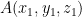$A({x_1},{y_1},{z_1})$ and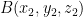$B({x_2},{y_2},{z_2})$ onto a line with direction cosines$l$,$m$,$n$.
 Solution: 5
Let us first consider a vector approach to this problem. The vector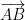$\overrightarrow {AB}$ can be written as$\overrightarrow {AB} = \left( {{x_2} - {x_1}} \right)\hat i + \left( {{y_2} - {y_1}} \right)\hat j + \left( {{z_2} - {z_1}} \right)\hat k$
A unit vector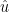$\hat u$ along the line with direction cosines$l$$m$$n$ will be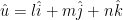$\hat u = l\hat i + m\hat j + n\hat k$
Therefore, the projected length of$\overrightarrow {AB}$ upon this line will be.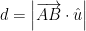$d = \left| {\overrightarrow {AB} \cdot \hat u} \right|$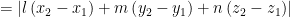$= \left| {l\left( {{x_2} - {x_1}} \right) + m\left( {{y_2} - {y_1}} \right) + n\left( {{z_2} - {z_1}} \right)} \right|$
This assertion can also be proved without resorting to the use of vectors. For this, we first understand the projection of a sequence of line segments on a given line.
Assume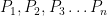${P_1},{P_2},{P_3}\ldots {P_n}$ to be$n$ points in space. The sum of projections of the sequence of segments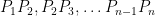${P_1}{P_2},{P_2}{P_3},\ldots {P_{n - 1}}{P_n}$ onto a fixed line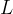$L$ will be the same as the projection of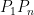${P_1}{P_n}$ onto$L$. This should be obvious from the following diagram:The projection of the segment${P_1}{P_n}$ onto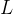$L$ is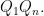${Q_1}{Q_n}.$ The sum of projections of segments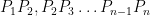${P_1}{P_2},{P_2}{P_3}\ldots {P_{n - 1}}{P_n}$ onto$L$ is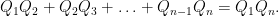${Q_1}{Q_2} + {Q_2}{Q_3} + \ldots + {Q_{n - 1}}{Q_n} = {Q_1}{Q_n}.$
We use this fact in our original problem as follows:The projection$d$ of$AB$ onto any line$L$ (with direction cosines say$l$$m$,$n$ ) will be sum of projections of$AC$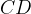$CD$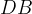$DB$ onto$L$. Since$AC$$CD$, and$DB$ are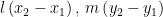$l\left( {{x_2} - {x_1}} \right),\,m\left( {{y_2} - {y_1}} \right)$ and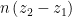$n\left( {{z_2} - {z_1}} \right)$ respectively, we get the total projection of$AB$ onto$L$ as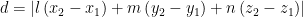$d = \left| {l\left( {{x_2} - {x_1}} \right) + m\left( {{y_2} - {y_1}} \right) + n\left( {{z_2} - {z_1}} \right)} \right|$